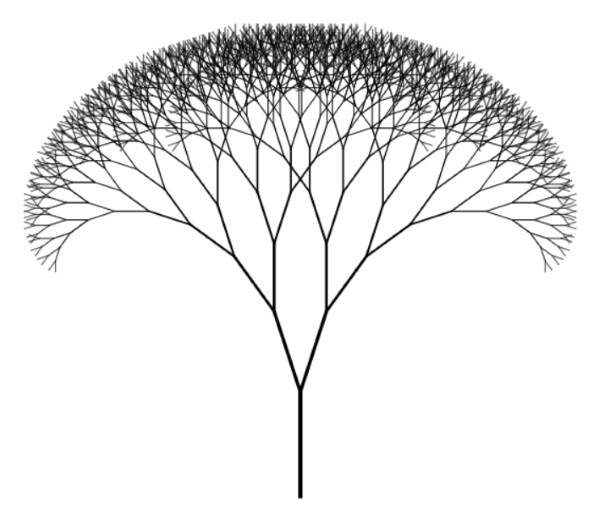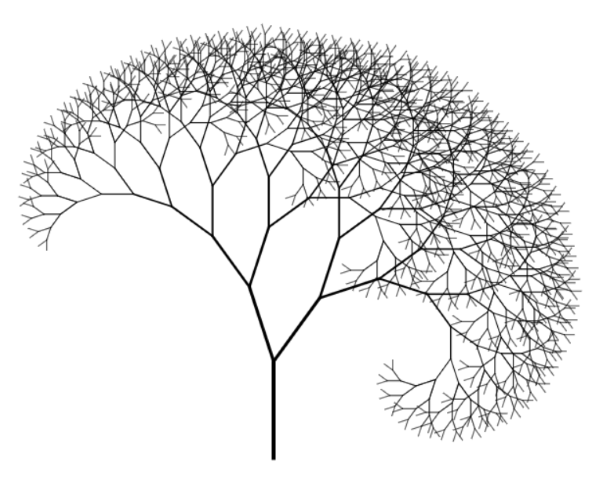A fractal tree drawn with PureScript

Last week, I attended Mathias Brandewinder's F# (and Clojure) dojo in Copenhagen, and had great fun drawing a fractal tree in F# together with other attendees. Afterwards, I started thinking that it'd be fairly easy to port the F# code to Haskell, but then I reconsidered. The combination of Haskell, Windows, and drawing sounded intimidating. This seemed a good opportunity to take PureScript for a spin, because that would enable me to draw the tree on an HTML canvas.

In case you're wondering, a fractal tree is simply a tree that branches infinitely in (I suppose) a deterministic fashion. Here's an example of the output of the code of this article:This is my first attempt at PureScript, and I think I spent between five and ten hours in total. Most of them I used to figure out how to install PureScript, how to set up a development environment, and so on. All in all I found the process pleasing.

While it's a separate, independent language, PureScript is clearly a descendant of Haskell, and the syntax is similar.

### Separating data from effects #

In functional programming, you routinely separate data from effects. Instead of trying to both draw and calculate branches of a tree in a single operation, you figure out how to first define a fractal tree as data, and then subsequently you can draw it.

A generic binary tree is a staple of functional programming. Here's one way to do it:

```data Tree a = Leaf a | Node a (Tree a) (Tree a)
```

Such a tree is either a leaf node with a generically typed value, or an (intermediate) node with a value and two branches, which are themselves (sub)trees.

In a sense, this definition is an approximation, because a 'real' fractal tree has no leafs. In Haskell you can easily define infinite trees, because Haskell is lazily evaluated. PureScript, on the other hand, is eagerly evaluated, so infinite recursion would require jumping through some hoops, and I don't think it's important in this exercise.

While the above tree type can contain values of any type, in this exercise, it should contain line segments. One way to do this is to define a `Line` record type:

```data Line = Line {
x :: Number,
y :: Number,
angle :: Number,
length :: Number,
width :: Number }
```

This is a type with five labelled values, all of them numbers. `x` and `y` are coordinates for the origin of the line, `angle` defines the angle (measured in radians) from the origin, and `length` the length of the line. In a similarly obvious vein, `width` denotes the width of the line, although this data element has no impact on the calculation of the tree. It's purely a display concern.

Given the first four numbers in a `Line` value, you can calculate the endpoint of a line:

```endpoint :: forall r.
{ x :: Number
, y :: Number
, angle :: Number
, length :: Number
| r }
-> Tuple Number Number
endpoint line =
-- Flip the y value because Canvas coordinate system points down from upper
-- left corner
Tuple
(line.x + line.length * cos line.angle)
(-(-line.y + line.length * sin line.angle))
```

This may look more intimidating than it really is. The first seven lines are simply the (optional) type declaration; ignore that for a minute. The function itself is a one-liner, although I've formatted it on several lines in order to stay within an 80 characters line width. It simply performs a bit of trigonometry in order to find the endpoint of a line with an origin, angle, and length. As the code comment states, it negates the `y` value because the HTML canvas coordinate system points down instead of up (larger `y` values are further towards the bottom of the screen than smaller values).

The function calculates a new set of coordinates for the endpoint, and returns them as a tuple. In PureScript, tuples are explicit and created with the `Tuple` data constructor.

In general, as far as I can tell, you have less need of tuples in PureScript, because instead, you have row type polymorphism. This is still a new concept to me, but as far as I can tell, it's a sort of static duck typing. You can see it in the type declaration of the `endpoint` function. The function takes a `line` argument, the type of which is any record type that contains `x`, `y`, `angle`, and `length` labels of the `Number` type. For instance, as you'll soon see, you can pass a `Line` value to the `endpoint` function.

### Creating branches #

In a fractal tree, you calculate two branches for any given branch. Typically, in order to draw a pretty picture, you make the sub-branches smaller than the parent branch. You also incline each branch an angle from the parent branch. While you can hard-code the values for these operations, you can also pass them as arguments. In order to prevent an explosion of primitive function arguments, I collected all such parameters in a single data type:

```data FractalParameters = FractalParameters {
leftAngle :: Number,
rightAngle :: Number,
shrinkFactor :: Number }
```

This is a record type similar to the `Line` type you've already seen.

When you have a `FractalParameters` value and a (parent) line, you can calculate its branches:

```createBranches :: FractalParameters -> Line -> Tuple Line Line
createBranches (FractalParameters p) (Line line) =
Tuple left right
where
Tuple x y = endpoint line
left = Line {
x: x,
y: y,
angle: pi * (line.angle / pi + p.leftAngle),
length: (line.length * p.shrinkFactor),
width: (line.width * p.shrinkFactor) }
right = Line {
x: x,
y: y,
angle: pi * (line.angle / pi - p.rightAngle),
length: (line.length * p.shrinkFactor),
width: (line.width * p.shrinkFactor) }
```

The `createBranches` function returns a tuple of `Line` values, one for the left branch, and one for the right branch. First, it calls `endpoint` with `line`. Notice that `line` is a `Line` value, and because `Line` defines `x`, `y`, `angle`, and `length` labels, it can be used as an input argument. This type-checks because of row type polymorphism.

Given the endpoint of the parent line, `createBranches` then creates two new `Line` values (`left` and `right`) with that endpoint as their origins. Both of these values are modified with the `FractalParameters` argument, so that they branch off to the left and the right, and also shrink in an aesthetically pleasing manner.

### Creating a tree #

Now that you can calculate the branches of a line, you can create a tree using recursion:

```createTree :: Int -> FractalParameters -> Line -> Tree Line
createTree depth p line =
if depth <= 0
then Leaf line
else
let Tuple leftLine rightLine = createBranches p line
left  = createTree (depth - 1) p leftLine
right = createTree (depth - 1) p rightLine
in Node line left right
```

The `createTree` function takes a `depth` argument, which specifies the depth (or is it the height?) of the tree. The reason I called it `depth` is because `createTree` is recursive, and `depth` controls the depth of the recursion. If `depth` is zero or less, the function returns a `Leaf` node containing the input `line`.

Otherwise, it calls `createBranches` with the input `line`, and recursively calls `createTree` for each of these branches, but with a decremented `depth`. It then returns a `Node` containing the input `line` and the two sub-trees `left` and `right`.

This implementation isn't tail-recursive, but the above image was generated with a recursion depth of only 10, so running out of stack space wasn't my biggest concern.

### Drawing the tree #

With the `createTree` function you can create a fractal tree, but it's no fun if you can't draw it. You can use the `Graphics.Canvas` module in order to draw on an HTML canvas. First, here's how to draw a single line:

```drawLine :: Context2D -> Line -> Eff (canvas :: CANVAS) Unit
drawLine ctx (Line line) = do
let Tuple x' y' = endpoint line
void \$ strokePath ctx \$ do
void \$ moveTo ctx line.x line.y
void \$ setLineWidth line.width ctx
void \$ lineTo ctx x' y'
closePath ctx
```

While hardly unmanageable, I was surprised that I wasn't able to find a pre-defined function that would let me draw a line. Perhaps I was looking in the wrong place.

With this helper function, you can now draw a tree using pattern matching:

```drawTree :: Context2D -> Tree Line -> Eff (canvas :: CANVAS) Unit
drawTree ctx (Leaf line) = drawLine ctx line
drawTree ctx (Node line left right) = do
drawLine ctx line
drawTree ctx left
drawTree ctx right
```

If the input tree is a `Leaf` value, the first line matches and the function simply draws the line, using the `drawLine` function.

When the input tree is a `Node` value, the function first draws the `line` associated with that node, and then recursively calls itself with the `left` and `right` sub-trees.

### Execution #

The `drawTree` function enables you to draw using a `Context2D` value, which you can create from an HTML canvas:

```mcanvas <- getCanvasElementById "canvas"
let canvas = unsafePartial (fromJust mcanvas)
ctx <- getContext2D canvas
```

From where does the `"canvas"` element come? Ultimately, PureScript compiles to JavaScript, and you can put the compiled script in an HTML file together with a `canvas` element:

```<body>
<canvas id="canvas" width="800" height="800"></canvas>
<script src="index.js" type="text/javascript"></script>
</body>```

Once you have a `Context2D` value, you can draw a tree. Here's the whole entry point, including the above canvas-finding code:

```main :: Eff (canvas :: CANVAS) Unit
main = do
mcanvas <- getCanvasElementById "canvas"
let canvas = unsafePartial (fromJust mcanvas)
ctx <- getContext2D canvas

let trunk = Line
{ x: 300.0, y: 600.0, angle: (pi / 2.0), length: 100.0, width: 4.0 }
let p = FractalParameters
{ leftAngle: 0.1, rightAngle: 0.1, shrinkFactor: 0.8 }
let tree = createTree 10 p trunk
drawTree ctx tree
```

After it finds the `Context2D` value, it hard-codes a `trunk` `Line` and a set of `FractalParameters`. From these, it creates a tree of size 10 and draws the tree in the beginning of this article.

You can fiddle with the parameters to your liking. For example, you can make the right angle wider than the left angle:

```let p = FractalParameters
{ leftAngle: 0.1, rightAngle: 0.2, shrinkFactor: 0.8 }
```

This produces an asymmetric tree:In order to compile the code, I used this command:

`\$ pulp browserify -t html/index.js`

This compiles the PureScript code to a single `index.js` file, which is output to the `html` directory. This directory also contains the HTML file with the canvas.

You can find the entire PureScript file in this Gist.

### Summary #

It was fun to try PureScript. I've been staying away from JavaScript-based development for many years now, but if I ever have to do some client-side development, I may consider it. So far, I've found that PureScript seems viable for drawing. How good it is if you need to interact with 'normal' web-pages or SPAs, I don't know (yet).

If you have some experience with Haskell, it looks like it's easy to get started with PureScript.

### Wish to comment?

You can add a comment to this post by sending me a pull request. Alternatively, you can discuss this post on Twitter or somewhere else with a permalink. Ping me with the link, and I may respond.

#### Published

Tuesday, 06 June 2017 08:10:00 UTC

#### Tags

"Our team wholeheartedly endorses Mark. His expert service provides tremendous value."
Hire me!
Published: Tuesday, 06 June 2017 08:10:00 UTC